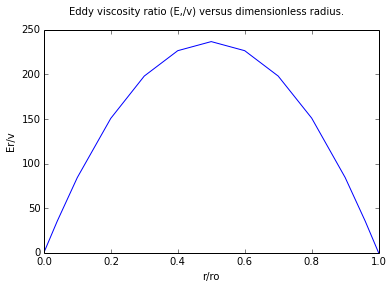# Chapter 6 : Turbulent Flow¶

### Example 6.1 - Page No :200¶

In :
import math

# Variables
# given
q = 50.;        			    #[gal/min] - volumetric flow rate
d = 2.067/12;  	    		    #[ft] - diameter
A = 0.02330;  		             #[ft**2] - flow area
p = 0.99568*62.43;  			 #[lb/ft**3] - density of water at 86degF
mu = 0.8007*6.72*10**-4;  		 #[lb/ft*sec] - viscosity of water at 86degF
u = q/(60.*7.48*A);

# Calculations
# using the formula Nre = d*u*p/mu;
Nre = round((d*u*p)/mu,-2);

# Results
print "Nre = ",Nre
print "Hence the flow is turbulent. Note also that Nre is dimensionless";

Nre =  95100.0
Hence the flow is turbulent. Note also that Nre is dimensionless


### Example 6.2 - Page No :202¶

In :
# Variables
# given
p = 0.99568*62.43;  			 #[lb/ft**3] - density of water at 86degF
mu = 0.8007*6.72*10**-4;  		 #[lb/ft*sec] - viscosity of water at 86degF
u = 4.78;  			             #[ft/sec] - free stream velocity
Nre = 5.*10**5;  			     # the lower limit for the transition reynolds number range is substituted

# Calculations
x = (Nre*mu)/(p*u);

# Results
print "x = %.1f"%x
print "Thus the transition could star at about %.2f ft. \
\nThe reynolds number at the  upper end of the transition range is %.0e .\
\nThe value of x at this location is ten times then the value obtained above i.e %.1f ft"%(x,Nre*10,x*10)

x = 0.9
Thus the transition could star at about 0.91 ft.
The reynolds number at the  upper end of the transition range is 5e+06 .
The value of x at this location is ten times then the value obtained above i.e 9.1 ft


### Example 6.3 - Page No :212¶

In :
# Variables
# given
t = [0, 0.01, 0.02, 0.03, 0.04, 0.05, 0.06, 0.07, 0.08, 0.09, 0.10, 0.11, 0.12];
Ux = [3.84, 3.50, 3.80, 3.60, 4.20, 4.00, 3.00, 3.20, 3.40,  3.00, 3.50, 4.30, 3.80];
Uy = [0.43, 0.21, 0.18, 0.30, 0.36, 0.28, 0.35, 0.27, 0.21, 0.22, 0.23, 0.36, 0.35];
Uz = [0.19, 0.16, 0.17, 0.13, 0.09, 0.10, 0.16, 0.15, 0.13, 0.18, 0.17, 0.18, 0.17];
# using the formula AREA = [deltat/2]*[U1+U13+2*[U2+U3+U4+U5+U6+U7+U8+U9+U10+U11+U12]]
# for Uxmean
deltat = 0.01;
T = t-t;

# Calculation and Results
AREA = (deltat/2)*(Ux+Ux+2*(Ux+Ux+Ux+Ux+Ux+Ux+Ux+Ux+Ux+Ux+Ux));
Uxmean = AREA/T;
print "Uxmean = %.2f m s**-1"%Uxmean

# for Uymean
deltat = 0.01;
AREA = (deltat/2)*(Uy+Uy+2*(Uy+Uy+Uy+Uy+Uy+Uy+Uy+Uy+Uy+Uy+Uy));
Uymean = AREA/T;
print "Uymean = %.2f m s**-1"%Uymean

# for Uzmean
AREA = (deltat/2)*(Uz+Uz+2*(Uz+Uz+Uz+Uz+Uz+Uz+Uz+Uz+Uz+Uz+Uz));
Uzmean = AREA/T;
print "Uzmean = %.2f m s**-1"%Uzmean
U = (Uxmean**2+Uymean**2+Uzmean**2)**(1./2);
print "U = %.3f m s**-1"%U

Uxmean = 3.61 m s**-1
Uymean = 0.28 m s**-1
Uzmean = 0.15 m s**-1
U = 3.624 m s**-1


### Example 6.4 Page no : 218¶

In :
import math

# Variables
#Following values are taken from example 6.3
U_dash_z2 = 8.917*10**-4   #m**2s**-2  square velocity
Uz2 = .02340               #m**2s**-2
U_dash_x2 = 8.947 * 10**-4 #m**2s**-2
Ux22 = .02409              #m**2s**-2
U = 3.634
U2x = 3.63                  #m**2s**-2
U2x2 = .41                  #m**2s**-2
Ix = 11.37                  #percent
U2y = .0288                 #m**2s**-2
U2y2 = .069                 #m**2s**-2
Iy = 1.92                   #percent
dt = .01108

# Calculation
rmsUz = math.sqrt(U_dash_z2)
rmsUx = math.sqrt(Uz2)
rmsUx2 = math.sqrt(U_dash_x2)
rmsUx2 = math.sqrt(Ux22)
I = 100*rmsUz/U
Ux = 3.84 - 3.61
Uy = .43 - .28
UxUy = Ux*Uy
ratio = dt/(U2x2*U2y2)

# Results
print "I = %.2f percent"%I
print "U'xU'y = %.4f m**2s**-2"%UxUy
print "The ratio = %.2f "%ratio

I = 0.82 percent
U'xU'y = 0.0345 m**2s**-2
The ratio = 0.39


### Example 6.5 - Page No :232¶

In :
%matplotlib inline

from numpy import *
from matplotlib.pyplot import *
import math

# Variables
# given
UzmaxbyU = 24.83;
roUbyv = 2312.;
Re = 100000.;

# using the formula Et/v = 95.5*((r/ro)/slope)-1
# from fig 6.6 at Re = 100000
rbyro = [0, 0.040, 0.100, 0.200, 0.300, 0.4, 0.5, 0.6, 0.7, 0.8, 0.9, 0.960, 1]
slope = [0, 0.105, 0.112, 0.126, 0.144, 0.168, 0.201, 0.252, 0.336, 0.503, 1.007, 2.517, 94.59]

# Calculations
Etbyv = zeros(13)
for i in range(1,13):
Etbyv[i] = 95.5*((rbyro[i])/slope[i])-1;

# Results
plot(rbyro,Etbyv);
suptitle("Eddy viscosity ratio (E,/v) versus dimensionless radius.")
xlabel("r/ro")
ylabel("Er/v")

show()

Populating the interactive namespace from numpy and matplotlib### Example 6.7 page no : 246¶

In :
import math

# variables
do = .0508
Uz = .8467                 # cyclohexane
aveP = 774.9               # the density of cyclohexane
u = 8.892*10**-4           # the viscosity
aveF = .00570

# Calculation
Nre = do*Uz*aveP/u
rw = (1./2)*aveP*(Uz**2)*aveF
U = math.sqrt(rw/aveP)

# for y = .001
y001 = (2.54*10**-5)*U*aveP/u

# Results
print "Nre = %.2e "%Nre
print "The wall shear stress rw = %.3f N m**-2"%rw
print "The friction velocity U* = %.5f m s*-1"%U
print "Universal velocity Distribution :"
print "for y = 0.001 = %d "%y001

Nre = 3.75e+04
The wall shear stress rw = 1.583 N m**-2
The friction velocity U* = 0.04520 m s*-1
Universal velocity Distribution :
for y = 0.001 = 1


### Example 6.8 Pageno :250¶

In :
import math

# variables
Cp = 4184.           # lg-K-=4184Jkg-K-*
P = 1000.            # g cm**-3
k = 0.628            # wm**-1
Nre = 1.2*10**5
v = 1*10**-6
do = 2*0.05

# calculation
alpha = k/(P*Cp)
qa = (1.7*10**4)/(P*Cp)
Uz = Nre*v/(do)
U = Uz*math.sqrt(.0045/2)
y = (5*v)/U

# Results
print "A = %.3e m**2s**-2"%alpha
print "The average velocity = %.2f m s**-1"%Uz
print "U* = %.5f m s**-1"%U
print "the value of y = %.3e cm"%y

A = 1.501e-07 m**2s**-2
The average velocity = 1.20 m s**-1
U* = 0.05692 m s**-1
the value of y = 8.784e-05 cm


### Example 6.9 - Page No :258¶

In :
# Variables
p = 0.84*62.4;  			 #[lbf/ft**3] - density
dP = 80.*144;  			 #[lbf/ft**2] - pressure
dz = 2000.;  			 #[ft] - length of pipe
gc = 32.174;  			 #[(lbm*ft)/(lbf*sec**2)] - gravitational conversion consmath.tant
dpbydz = -dP/dz;
do = 2.067/12;  			 #[ft]

# Calculations
U = 2000*(1./24)*(1./3600)*(42)*(1./7.48)*(1./0.02330);
# using the formula f = ((do/2)*(-dp/dz)*gc)/(p*(U)**2)
f = ((do/2)*(-dpbydz)*gc)/(p*(U)**2)

# Results
print "f = %.5f (dimensionless)"%f

f = 0.00979 (dimensionless)